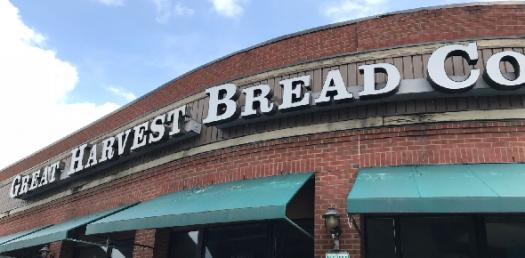# Week 2 Halliburton Ttp

103 Questions | Total Attempts: 347SettingsThis quiz will deal with a few different components in the study of sound, with specific reference to wave velocity, acoustic porosity, transmitters, Delta-T, P-wave, voltage, vertical resolution and electrode devices. See how much you know about it!

Related Topics
• 1.
What type of wave travels fastest through a formation?
• A.

Shear

• B.

Mud

• C.

Surface

• D.

Compressional

• 2.
Acoustic porosity services are NOTcapable of recording acoustic waves in
• A.

Air-drilled holes

• B.

Oil-based mud

• C.

Freshwater-based mud

• D.

Saltwater-based mud

• 3.
Which of the following is not considered a body wave?
• A.

Shear wave

• B.

Compressional wave

• C.

Stoneley wave

• 4.
What does Delta-T represent?
• A.

The time required for sound to travel from the transmitter to a receiver.

• B.

The time required for sound to travel through a one foot interval of formation.

• C.

The distance traveled by a direct wave from the transmitter through the formation and detected at the receiver.

• 5.
Which of the following is not a practical advantage of increasing offset between transmitter and receiver?
• A.

Potential for deeper depth of investigation beyond the altered zone

• B.

Enables accurate data acquisition in large diameter boreholes

• C.

Enables faster logging speeds

• 6.
What effect do slots cut into the tool housing have on tool mode waves?
• A.

Increased velocity

• B.

Decreased travel times

• C.

Increased travel times

• D.

Decreased velocity

• 7.
Compressional detla-T (Δtc) _____________ as formation porosity increases.
• A.

Remains constant

• B.

Decreases

• C.

Increases

• 8.
Select all of the following that are considered body waves..
• A.

Mud Waves

• B.

Compressional Waves

• C.

Stoneley Waves

• D.

Leaky Mode

• E.

Shear Waves

• 9.
Compressional detla-T (Δtc) is proportional to the reciprocal of P-wave velocity.
• A.

True

• B.

False

• 10.
Depth-derived borehole compensation used by the Long Spaced Sonic compensates for both tools tilt and washouts.
• A.

True

• B.

False

• 11.
Compressional wave velocity is a function of the elastic properties and bulk density of the medium through which it travels.
• A.

True

• B.

False

• 12.
The BCDT compensates for the effects of tool tilt and washouts.
• A.

True

• B.

False

• 13.
The distance between transmitter and receiver is known as:
• A.

Offset

• B.

Spacing

• C.

Arrival

• 14.
Acoustic porosity provides a measure of the effective porosity of the formation.
• A.

True

• B.

False

• 15.
A cycle skip is when P-wave first arrivals at two receivers are selected on different cycles of the waveform.
• A.

True

• B.

False

• 16.
Which of the following is the DFL's depth of investigation?.
• A.

24 inches

• B.

17 inches

• C.

12 inches

• D.

10 inches

• 17.
Select all the potential applications of the DFL measurement when combined with the HRID measurement.
• A.

Measure formation porosity

• B.

Qualitative estimation of permeability

• C.

Measure resistivity of the drilling fluid

• D.

Determination of flushed zone water saturation

• E.

Estimation of diameter of invasion

• 18.
The physical law which states that resistivity is directly proportional to voltage and inversely proportional to current is:
• A.

Ampere’s law

• B.

Ohm’s law

• C.

• D.

Mitchell’s law

• 19.
On the DFL, bucking current (BC) is:
• A.

Emitted from the Ao electrode and returns to the upper and lower A1 electrodes.

• B.

Emitted from the Ao electrode and returns to the current return electrode.

• C.

Emitted from the current return electrode and returns to the Ao electrode.

• D.

Emitted from the upper and lower A1 electrodes and returns to the Ao electrode.

• 20.
On the DFL, measure current (MC) is:
• A.

Emitted from the A0 electrode and returns to the upper and lower A1 electrodes.

• B.

Emitted from the current return electrode and returns to the A0 electrode.

• C.

Emitted from the A0 electrode and returns to the current return electrode.

• D.

Emitted from the upper and lower A1 electrodes and returns to the A0 electrode.

• 21.
Measure voltage (VE) and bucking voltage (VB) are measured between:
• A.

The Ao electrode and the current return electrode

• B.

Monitor electrodes positioned along the HRID sonde assembly

• C.

The Ao electrode and the upper and lower A1 electrodes

• D.

Receiver coils within the HRID sonde assembly

• 22.
What is the vertical resolution of the DFL?
• A.

< 17 inches

• B.

< 10 inches

• C.

< 12 inches

• D.

< 5 inches

• 23.
An electrode device such as the DFL operates by:
• A.

Injecting a voltage into the formation and measuring a current between two electrodes.

• B.

Inducing a current into the formation and measuring a voltage between two receiver coils.

• C.

Injecting a current into the formation and measuring a voltage drop between two electrodes.

• D.

Inducing a voltage into the formation and measuring a current between two receiver coils.

• 24.
A primary objective of logging the Digitally Focused Laterolog (DFL) is to determine:
• A.

Formation water resistivity (Rw)

• B.

True resistivity of the uninvaded formation (Rt)

• C.

Mud filtrate resistivity (Rmf)

• D.

Flushed zone resistivity (Rxo)

• 25.
Which of the following is a true statement?
• A.

The DSNT is used to help estimate the volume of shale in a formation when combined with density porosity or sonic porosity.

• B.

The DSNT is used to determine a value for true resitivity of a formation.

• C.

The DSNT is often used as a quantitative estimate of permeability.